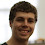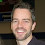## Saturday, March 20, 2010

### Code Kata and Project Euler

Recently, I've been thinking about the concept of Code Kata and the personal improvement that this type of practice can bring.  I started thinking of types of exercises that I could do to utilize this concept.  After looking around on the web, I landed on projecteuler.net and found that the problems listed seemed to fit my agenda perfectly.

For those of you who don't know, "Project Euler is a series of challenging mathematical/computer programming problems..." (ProjectEuler.net).  The mathematical nature of these problems make them a great fit for the functional paradigm.

Here's the first problem:

"Add all the natural numbers below one thousand that are multiples of 3 or 5."  Additional details include the following simple example: "If we list all the natural numbers below 10 that are multiples of 3 or 5, we get 3, 5, 6 and 9. The sum of these multiples is 23."

With the help of the simple example, I created a test that looked like this:

```
module Problem1Tests

open NUnit.Framework

[<TestFixture>]
type FindPrimes2__When_Getting_Sum_Of_Multiples_Of_3_And_5_to_a_max_number_of_10 () =
[<Test>]
member this.should_return_sum_of_23 () =
let result = Problem1.GetSumOfMultiplesOf3And5(10)
Assert.AreEqual(23, result)
```

After a few refactoring sessions, my final code looked like this:

```
module Problem1

let GetSumOfMultiplesOf3And5 max =
seq{3..max-1} |> Seq.fold(fun acc number ->
(if (number % 3 = 0 || number % 5 = 0) then
acc + number else acc)) 0
```

Have you found good Code Kata exercises?  If so, I'd love to see them.  Also, let me know if you have a better solution for the problem above.

1.Well, IEnumerable does have some overhead. One way to reduce this might be to Seq.unfold with your filter. As you only need the last value and sum, another way to speed this up would be to take out all of the sequence stuff.

let sumMultipleOf3or5UpTo max =
let rec nextMultipleOf3or5 x =
match x + 1 with
| n when n % 3 = 0 || n % 5 = 0 -> n
| n -> nextMultipleOf3or5 n
let rec loop last max acc =
let next = nextMultipleOf3or5 last
if (next >= max) then acc else loop next max (acc + next)
loop 1 max 0

Admittedly not as pretty, but probably a bit faster.

Best,
-Rick

2.Thanks, Dan. I've been looking for something like this.

3.Looks very similar in Ruby:

def sum_mults_of_3_or_5_below(max)
(1...max).to_a.
select { |i| i % 3 == 0 || i % 5 == 0 }.
inject(0) { |sum, i| sum + i }
end

4.This comment has been removed by the author.

5.Here's my solution. It's the result of imagining the successive increments as steps up to MAX so that the steps form a diagonal line through the shape that would be formed if all steps were as a tall as the tallest step. This doesn't deeply use F#, but it avoids unnecessary computation.

let sumSteppedIncrements x max =
let divResult =
if max % x > 0 then
max / x
else
// All numbers must be less than the max,
// remove one to ignore the multiple of x that is less than max.
max / x - 1
// We count the first step when the sum is zero.
let zerothStep = 1
let numSteps = divResult + zerothStep

((divResult * x) * numSteps) / 2

let max = 1000

let threesAnswer = sumSteppedIncrements 3 max;;
let fivesAnswer = sumSteppedIncrements 5 max;;

// Since 3 * 5 = 15, we have counted multiples of fifteen twice.
// We need the sum of the those numbers so we can correct this by subtraction.
let fifteensAnswer = sumSteppedIncrements (3 * 5) max;;

A better formatted source file is here.

6.Also, if you care to, please tell me how my F# sucks. :) dmohl and rick, thanks for facilitating so much discussion.

7.I realized later that my sample wasn't really in the spirit of code kata because it was oriented more to math than to code. Sorry to have added some noise.

8.Why complicating lives with F# ?
// javascript
function euler ( x, y) {
var rez = 0 ;
while ( x-- > y) {
if (x % y == 0)
rez += x
}
return rez ;
}

euler(1000,5) + euler( 1000, 3 )

Also, why "oring" (aka ||) inside the loop ?

--DBJ

9.I realized later that my sample wasn't really in the spirit of code kata because it was oriented more to math than to code. Sorry to have added some noise.

10.Here's my solution. It's the result of imagining the successive increments as steps up to MAX so that the steps form a diagonal line through the shape that would be formed if all steps were as a tall as the tallest step. This doesn't deeply use F#, but it avoids unnecessary computation.

let sumSteppedIncrements x max =
let divResult =
if max % x > 0 then
max / x
else
// All numbers must be less than the max,
// remove one to ignore the multiple of x that is less than max.
max / x - 1
// We count the first step when the sum is zero.
let zerothStep = 1
let numSteps = divResult + zerothStep

((divResult * x) * numSteps) / 2

let max = 1000

let threesAnswer = sumSteppedIncrements 3 max;;
let fivesAnswer = sumSteppedIncrements 5 max;;

// Since 3 * 5 = 15, we have counted multiples of fifteen twice.
// We need the sum of the those numbers so we can correct this by subtraction.
let fifteensAnswer = sumSteppedIncrements (3 * 5) max;;

A better formatted source file is here.

11.Well, IEnumerable does have some overhead. One way to reduce this might be to Seq.unfold with your filter. As you only need the last value and sum, another way to speed this up would be to take out all of the sequence stuff.

let sumMultipleOf3or5UpTo max =
let rec nextMultipleOf3or5 x =
match x + 1 with
| n when n % 3 = 0 || n % 5 = 0 -> n
| n -> nextMultipleOf3or5 n
let rec loop last max acc =
let next = nextMultipleOf3or5 last
if (next >= max) then acc else loop next max (acc + next)
loop 1 max 0

Admittedly not as pretty, but probably a bit faster.

Best,
-Rick

12.http://dbj.org/dbj/?p=608

PS: make no mistake, I think F# is better than C#

13.I think there are strong cases for using F# for various tasks. http://msdn.microsoft.com/en-us/fsharp/gg634701 goes into some of "the benefits of F# for enterprise scenarios". That said, I don't really see F# as being a language to rule them all. I personally really like the combination of JavaScript on the client and F# on the server.